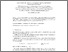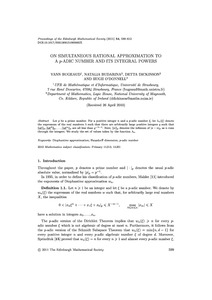# On simultaneous rational approximation to a p-adic number and its integral powers

Bugeaud, Yann and Budarina, Natalia and Dickinson, Detta and O'Donnell, Hugh (2011) On simultaneous rational approximation to a p-adic number and its integral powers. Proceedings of the Edinburgh Mathematical Society, 54 (3). pp. 599-612. ISSN 0013-0915Previewmore...Add this article to your Mendeley library

## Abstract

Let p be a prime number. For a positive integer n and a p -adic number ξ , let λ n ( ξ ) denote the supremum of the real numbers λ such that there are arbitrarily large positive integers q such that qξ p , qξ 2 p ,..., qξ n p are all less than q − λ − 1 . Here, x p denotes the infimum of | x − n | p as n runs through the integers. We study the set of values taken by the function λ n

Item Type: Article Diophantine approximation; Hausdorff dimension; p-adic number; Faculty of Science and Engineering > Mathematics and Statistics 4836 https://doi.org/10.1017/S001309151000060X Dr. Detta Dickinson 19 Mar 2014 15:10 Proceedings of the Edinburgh Mathematical Society Cambridge University Press (CUP) YesItem control page